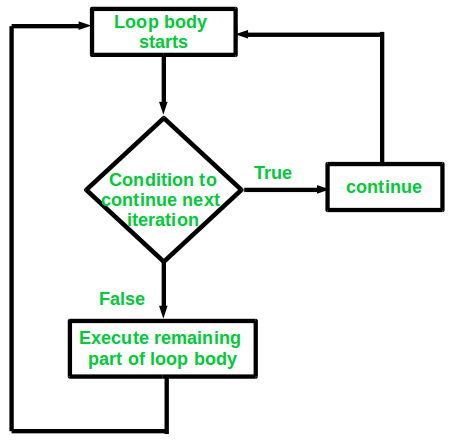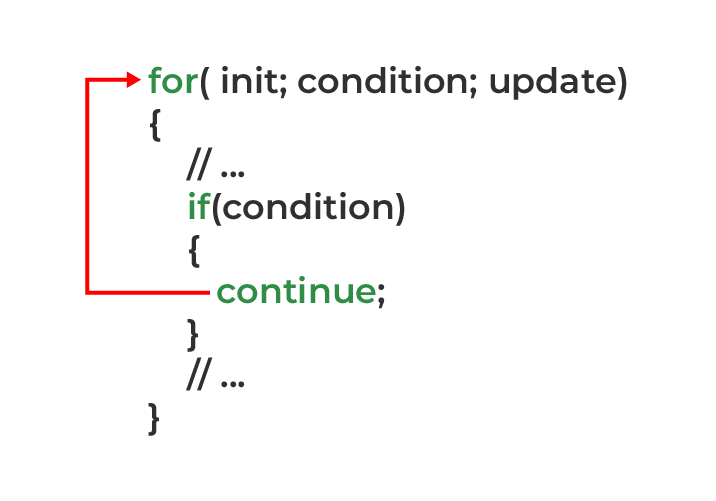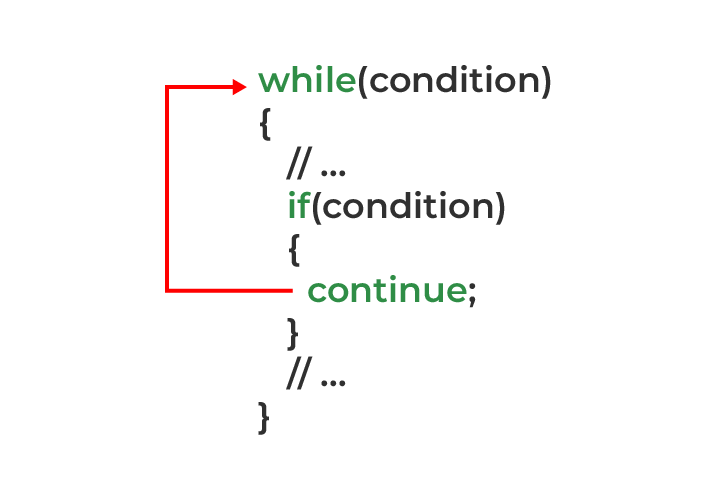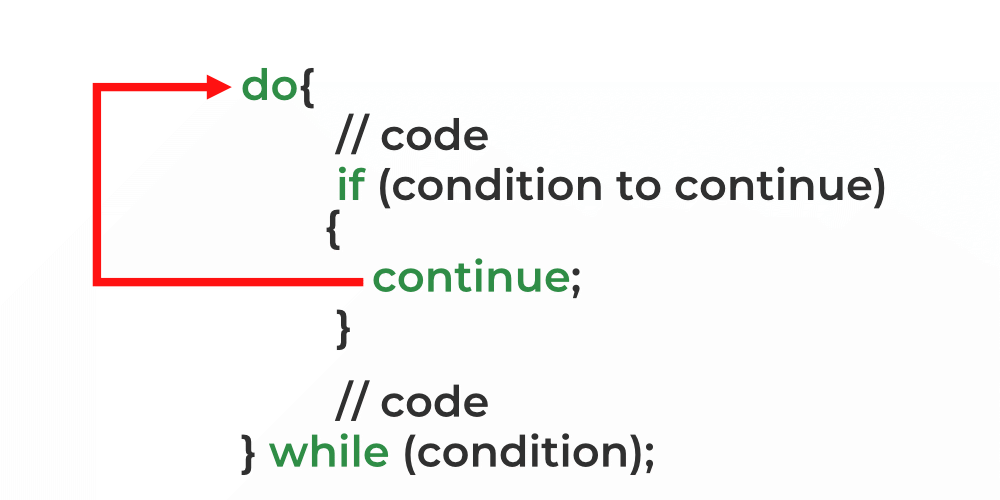Open In App

# continue Statement in C++

C++ continue statement is a loop control statement that forces the program control to execute the next iteration of the loop. As a result, the code inside the loop following the continue statement will be skipped and the next iteration of the loop will begin.

Syntax:

`continue;`Flowchart of continue Statement in C++

Example: Consider the situation when you need to write a program that prints numbers from 1 to 10 but not 4. It is specified that you have to do this using loop and only one loop is allowed to use. Here comes the usage of the continue statement. What we can do here is we can run a loop from 1 to 10 and every time we have to compare the value of the iterator with 4. If it is equal to 4 we will use the continue statement to continue to the next iteration without printing anything otherwise we will print the value.

## Continue Statement with for Loop

If continue is used in a for loop, the control flow jumps to the test expression after skipping the current iteration.Continue statement in for loop

Example:

## C++

 `// C++ program to explain the use``// of continue statement with for loop`` ` `#include ``using` `namespace` `std;`` ` `int` `main()``{``    ``// loop from 1 to 10``    ``for` `(``int` `i = 1; i <= 10; i++) {`` ` `        ``// If i is equals to 4,``        ``// continue to next iteration``        ``// without printing``        ``if` `(i == 4)``            ``continue``;`` ` `        ``else``            ``// otherwise print the value of i``            ``cout << i << ``" "``;``    ``}`` ` `    ``return` `0;``}`

Output

`1 2 3 5 6 7 8 9 10 `

The continue statement can be used with any other loop also like while or do while in a similar way as it is used with for loop above.

## Continue Statement with While Loop

With continue, the current iteration of the loop is skipped, and control flow resumes in the next iteration of while loop.The continue statement in while loop

Example:

## C++

 `// C++ program to explain the use``// of continue statement with while loop`` ` `#include ``using` `namespace` `std;`` ` `int` `main()``{``    ``int` `i = 0;``    ``// loop from 1 to 10``    ``while` `(i < 10) {``          ``i++;``        ``// If i is equals to 4,``        ``// continue to next iteration``        ``// without printing``        ``if` `(i == 4) {``            ``continue``;``        ``}`` ` `        ``else` `{``            ``// otherwise print the value of i``            ``cout << i << ``" "``;``        ``}``    ``}`` ` `    ``return` `0;``}`

Output

`1 2 3 5 6 7 8 9 10 `

## Continue Statement with do-while Loop

In do-while loop, the condition is checked after executing the loop body. When a continue statement is encountered in the do-while loop, the control jumps directly to the condition checking for the next loop, and the remaining code in the loop body is skipped.The continue statement in do-while loop

Example:

## C++

 `// C program to explain the use``// of continue statement with while loop`` ` `#include ``using` `namespace` `std;`` ` `int` `main()``{`` ` `    ``int` `i = 0;``    ``// loop from 1 to 10``    ``do` `{`` ` `        ``// If i is equals to 4,``        ``// continue to next iteration``        ``// without printing``        ``i++;`` ` `        ``if` `(i == 4) {``            ``continue``;``        ``}``        ``else` `{``            ``// otherwise print the value of i``            ``cout << i << ``' '``;``        ``}`` ` `    ``} ``while` `(i < 10);``    ``return` `0;``}`

Output

`1 2 3 5 6 7 8 9 10 `

Here, 4 is not printed as a continue statement is encountered in the case of i = 4.

Note: In while and do-while loop, we updated the loop variable i before the continue statement or else we will keep encountering continue before updating the loop variable creating an infinite loop.

## Continue Statement with Nested Loops

Using continue, you skip the current iteration of the inner loop when using nested loops.

Example:

## C++

 `// C++ program to explain the use``// of continue statement with nested loops`` ` `#include ``using` `namespace` `std;`` ` `int` `main()``{`` ` `    ``for` `(``int` `i = 1; i <= 2; i++) {``        ``for` `(``int` `j = 0; j <= 4; j++) {``            ``if` `(j == 2) {``                ``continue``;``            ``}``            ``cout << j << ``" "``;``        ``}``        ``cout << endl;``    ``}`` ` `    ``return` `0;``}`

Output

```0 1 3 4
0 1 3 4 ```

Continue skips the current iteration of the inner loop when it executes in the above program. As a result, the program is controlled by the inner loop update expression. In this way, 2 is never displayed in the output.

## Continue Statement vs Break Statement

There are some differences between the continue and break statements which alter the normal flow of a program.

Exercise Problem: Given a number n, print a triangular pattern. We are allowed to use only one loop.

```Input: 7
Output:
*
* *
* * *
* * * *
* * * * *
* * * * * *
* * * * * * *```

This article is contributed by Harsh Agarwal. If you like GeeksforGeeks and would like to contribute, you can also write an article using write.geeksforgeeks.org or mail your article to review-team@geeksforgeeks.org. See your article appearing on the GeeksforGeeks main page and help other Geeks.